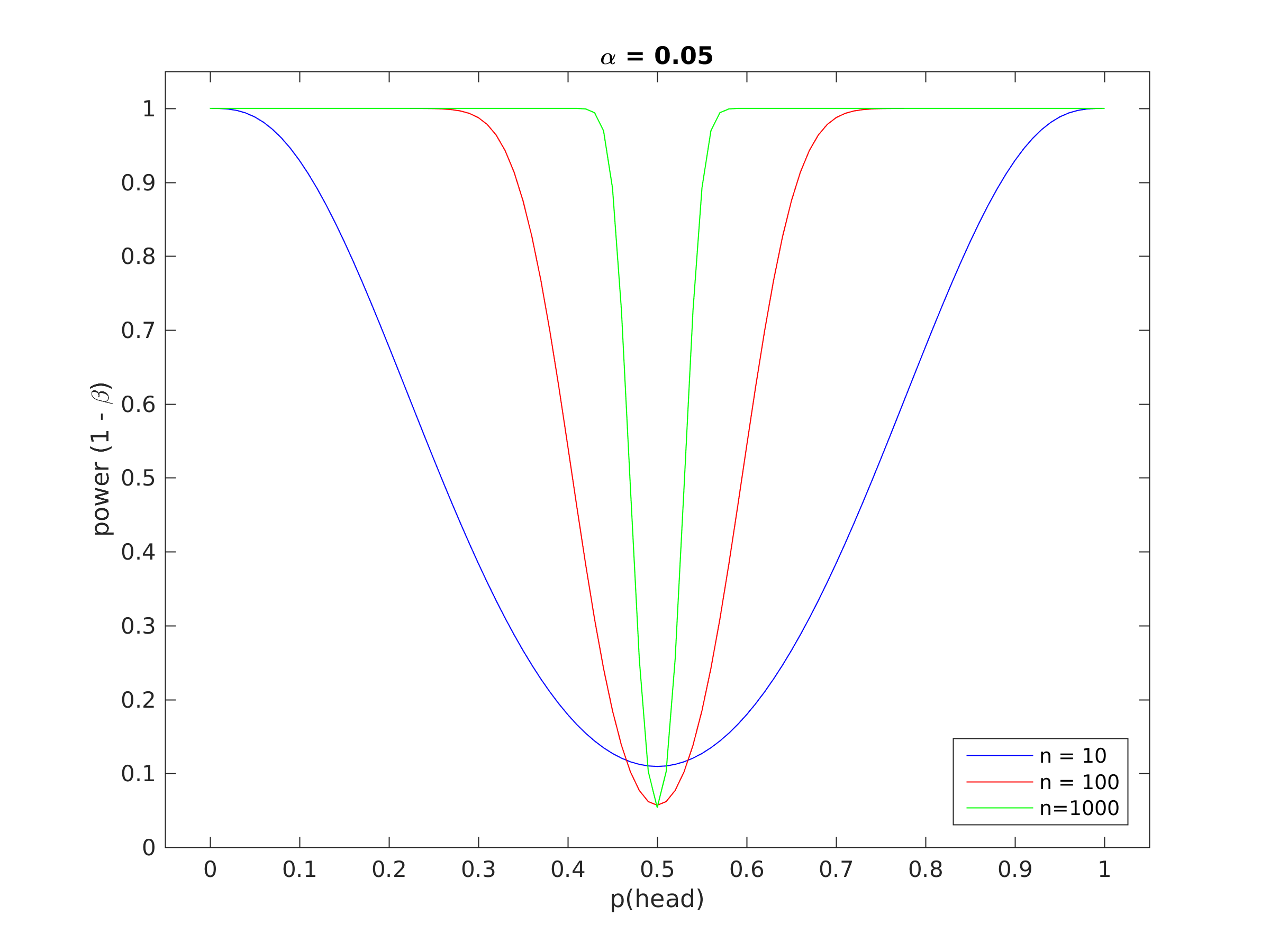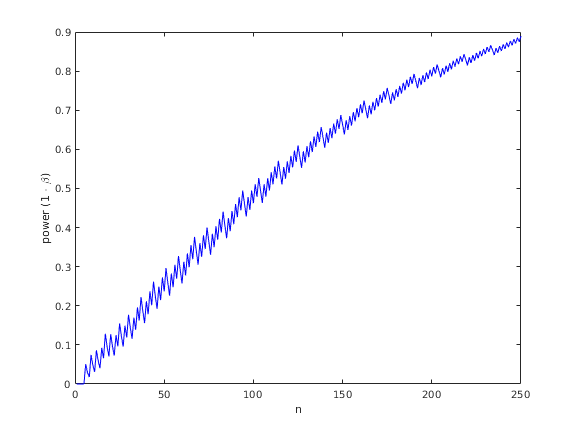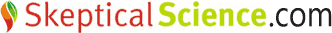# What Does Statistically Significant Actually Mean?

Used correctly, Null Hypothesis Statistical Testing (NHST) provides a valuable sanity check in science, requiring scientists to question the support their theories receive from the data, such that they only proceed with their research hypothesis if it can overcome this (often minimal) hurdle.  This enforces an element of healthy self-skepticism that helps science to be self-correcting in the long term.  Unfortunately, however, statistical hypothesis testing is widely misunderstood amongst the general public, working scientists and even professors of statistics .  It isn't unduly surprising then, that misunderstandings of statistical significance have cropped up rather frequently in the climate debate.  The aim of this post is to give an idea of what statistical significance actually means, and more importantly, what it doesn't mean and why this should matter.

### The Basic Null Hypothesis Statistical Testing Recipe

Flipping a coin is the traditional way of deciding between two arbitrary options, for instance which side should have the option of batting or fielding first in a game of cricket.  A classic example of statistical hypothesis testing is deciding whether a coin is fair (the probability of a head is the same as that of a tail) or whether it is biased.  Say we observe the coin being flipped four times and it comes down heads each time.  The usual recipe for statistical hypothesis testing is to first state your null hypothesis (known as H0), which is generally taken to be the thing you don't want to be true.  Say we think the captain of the opposition is a cheat and he is using a biased coin to gain an unfair advantage.  In that case, our null hypothesis ought to be that we are wrong and that his coin is fair (i.e. q = p(head) = p(tail) = 0.5).

H0: The coin is fair, q = 0.5

We then state our experimental hypothesis, for which we want to provide support

H1: The coin is biased, q ≠ 0.5

We then need a test statistic, z, that we use to measure the outcome of the experiment.  In this case, we record the number of heads in n = 4 trials, so z = 4.

The next step is where the self-skepticism is introduced.  We begin by assuming that H0 is true and work out the probability of observing an outcome at least as extreme as that actually observed, and only proceed with H1 if we can show that the observations would be sufficiently unlikely if H0 were true.  In other words, we assume that we are wrong, at least until we can show that the observations are inconsistent with the null hypothesis.

The probability of a test statistic at least as extreme as that observed is called the "p-value".  In this case, there is no more extreme result than four heads in a row if you only flip the coin four times, so the p-value is just the probability of getting four heads in four flips

p = q × q × q × q = 0.54 = 0.0625

We then compare the p-value with a threshold α (commonly α = 0.05, such that the "level of significance", 1-α = 0.95), chosen before performing the experiment. If the p-value is less than α, then the observations would be considered unlikely if the null hypothesis were true, so we say "we reject the null hypothesis" and that there is "statistically significant" evidence that the coin is biased.  If on the other hand, the p-value is greater than α, we say "we fail to reject the null hypothesis" and that the evidence for the coin being biased is "statistically insignificant".  In this case, the p-value is a bit larger than α, so we do not have statistically significant evidence for the coin being biased (and hence should not accuse the opposition captain of being a cheat!).

## So What Does This Tell Us?

Possibly very little.  There are two outcomes of the test, so lets consider them in turn:

### "We are Able to Reject the Null Hypothesis"

If the p-value is less than α, and we "reject the null hypothesis", we shouldn't rush to conclude that our research hypothesis is correct and that we have disproved the null hypothesis, as this XKCD cartoon neatly demonstrates:Here the frequentist (of which more later) statistician has performed the mechanics of the NHST correctly, but common sense tells us that it is very unlikely that he is correct in concluding that the Sun has gone nova!  So where has the test gone wrong?  In this case, the problem is that the significance level (1 - α) has been set much too low.  While the probability of a false alarm (H0) is small (1/36), the probability of it being set off under the alternate hypothesis H1 (the Sun has gone nova) is astronomically smaller.  The Sun has been shining for about 4,500,000,000 years, so the chances that it chose today to go nova is vanishingly small, so whenever the alarm goes off, it is almost certainly going to be a false alarm.  Statisticians traditionally use a significance level of 95% (α = 0.5) as a sensible default value, but unthinkingly using this default is a recipe for the kind of error illustrated by the XKCD cartoon.  In practice we should choose a significance level in accordance with our prior knowledge of the system or experiment, in this case, we would require a much higher significance level to believe an alarm was not a false alarm!  So if you see an NHST with a 95% significance level, you should question whether that threshold was reasonable, or whether it provides too low a hurdle for the conclusions drawn by the study.

### "We are Unable to Reject the Null Hypothesis"

If we are unable to reject the null hypothesis, there are at least two reasons why this might be the case.  The first, and most obvious, is that we fail to reject the null hypothesis simply because it happens to be true1!  The other alternative is that it is false, but we haven't collected enough observations to provide sufficient evidence that the coin is biased.  Say we have a double headed coin; before we have observed a single coin flip, we are in a state of complete ignorance regarding the bias of the coin, we have no evidence to go on.  If we observe an infinite number of coin flips and it comes down heads each time, we can be absolutely certain that the coin is double-headed.  As the number of flips observed increases and we get a head each time, we have stronger and stronger evidence for the bias of the coin.  The question is, how many flips do we need to observe to be confident that we would have rejected the null hypothesis when it actually is false.  To do this, we need to look at the statistical power of the test.

The "statistical power" of the test is the probability that the test will reject the null hypothesis when it is false, which statisticians express as 1 - β, where β is the probability of a false negative error (failing to reject H0 when it is false).  We can use statistical power to decide how much data we need to collect for us to be reasonably confident that if we fail to reject the null hypothesis it probably is because the null hypothesis is false.  So that we are not biased towards H0 or H1, we can set the desired statistical power such that the probability of a false negative error is the same as the probability of a false positive, i.e. β = α = 0.05.

[The calculation of statistical power is rather involved, so feel free to skip this bit]

The statistical power depends on the probability of a head if  the coin is biased (i.e. if H1 is true and H0 is false).  If the coin is double headed (q = 1) then we won't need a huge number of flips coming up heads each time to be confident that the coin is indeed biased.  However, if the coin is only slightly biased (say q = 0.6) then 40% of the flips will fall tails and it will be much less obvious that the coin is biased and more flips will be required.  Lets revise the example, such that

H1: The coin is biased, q = 0.6.

We can then compute the statistical power as a function of the number of flips, n, using the binomial distribution,which gives the probability of observing k heads in n flips, if the probability of observing a head on any given flip is p. The cumulative probability distribution is thenwhereis the largest integer greater than or equal to kFor a given number of flips, say n = 10, we can determine the range of values of k (the number of heads observed) for which we would be unable to reject H0 by finding the largest k such that F(k; 10, 0.5) < α/2 (as it is a two-sided test), in this case we find that we can reject H0 if k < 2, and by the symmetry of the binomial distribution for k >8.

Next we need to work out the probability that k lies in this region if H1 is true, i.e. p=0.6.   The probability of observing between 2 and 8 heads under H1 is then  β = F(2 ; 10, 0.6) - F(8 ; 10, 0.6) ≈ 0.9413, i.e. even if the coin is biased, we would be unable to reject H0 in about 94% of experiments, so we say the power of the test is (1 - β) = 0.0587, which is very low.  This means that while we were unable to reject H0, this isn't very surprising, because the same size is too small, so we shouldn't draw any strong conclusions.

Figure 1 shows a power curve for this example, i.e. a plot of the statistical power as a function of the actual probability of a head using a biased coin, clearly the more biased the coin, the fewer the number of observations we need to achieve satisfactory power.Figure 1.  Power curve for the coin tossing example.

The best thing to do would be to plot (1 - β) as a function of n, to find out how many flips we would require to achieve a reasonable level of statistical power, say (1 - β) = 0.8. Then we could be confident that if we were unable to reject the null hypothesis, it probably isn't because the sample size is too small. Figure 2 shows a plot of the statistical power as a function of the sample size, n, which suggests about 200 samples would be sufficient.Figure 2.  Statistical power as a function of sample size for the coin tossing example.

[rejoin the discussion here]

In the case of the test where we observed four heads in four flips, the power of the test is exactly zero, so we were always going to be unable to reject H0, no matter how biased the coin was, as we only observed four coin-flips.  So in this case, a lack of statistical significance doesn't mean that the coin is unbiased, just that there is insufficient evidence to rule out the possibility that it is unbiased!

You will notice that working out the statistical power, and hence the amount of data that needs to be collected, is a lot more complicated than performing the test itself.  Things are even more complicated if we look at a less straightforward problem, for instance whether there has been a warming trend in global mean surface temperatures (GMSTs) where such a calculation would be much less straightforward.  However, we only really need to worry about statistical power if we are arguing for rather than against the null hypothesis.  This is because in that case, we need to show that a failure to reject the null hypothesis is surprising, and that will only be true if the test has high statistical power.

## Why do we only say we are "Able/Unable to Reject H0"?

You might wonder why statisticians are so careful in describing the outcome of the test by saying only "we are able to reject the null hypothesis" or "we are unable to reject the null hypothesis" at a particular level of "significance", rather than "the probability that the null hypothesis is false is p".  Actually this happens quite a lot, it is known as the p-value fallacy. The problem stems from the definition of a probability.  There are two schools of statistics, the "Bayesians" who define a probability as a numeric measure of the plausibility of a proposition, and "frequentists", who define a probability as the long run frequency with which something occurs.  This causes a problem for frequentist NHSTs (Bayesian NHSTs have their problems as well) because whether a particular hypothesis (say H0) is true has no long run frequency, it is either true or it isn't which means that frequentists cannot meaningfully talk of the probability that H0 is true.  Unfortunately, this is exactly the form of answer we most want from a statistical test, which I think is probably why they are misinterpreted so frequently [sic].

## Hiatus, what Hiatus?

So how is this relevant to the climate debate?  You may have noticed that climate skeptics like to discuss the fact that global mean surface temperatures have not shown statistically significant warming since [insert cherry picked start date here] and conclude that this means that global warming has somehow stopped happening.  Regardless of the physics that suggests that this argument is at best questionable, does this argument have a solid statistical foundation?  No, not really, as in the coin-flipping test, if we fail to reject the null hypothesis (in this case that the underlying trend is flat) that can mean one of two things, either the null hypothesis is true and global warming has stopped, or that H0 is false, but we don't have enough data to confidently demonstrate that H0 is false.  The step that the skeptics have missed is to consider the statistical power of the hypothesis test.  As the expected rate of warming (about 0.2 degrees C per decade) is relatively small compared to the magnitude of the noise created by sources of internal variability, such as ENSO, we need quite a lot of data for the test to have sufficient power to reliably reject the null hypothesis when it is actually false.  This is why climatologists tend to use a long period of about 30 years to assess trends.

So next time a climate-skeptic tells you that there has been no statistically significant warming since [insert cherry picked start date here] and that there has been a hiatus in global warming, ask them if they have computed the statistical power of the test, and if they haven't ask them how they know that the lack of significance is not caused by having too little data, rather than because there actually has been a hiatus2.  They are also largely negating the value of NHSTs by adopting a null hypothesis that is the thing they are arguing for, rather than against, so it is (somewhat ironically) of little use in enforcing self-skepticism.

Of course, what they should do is to devise an NHST where the null hypothesis, H0, states that warming has continued at the same rate, and see if they can reject that...

### References

 Haller, H., & Krauss, S. (2002). Misinterpretations of significance: A problem students share with their teachers? Methods of Psychological Research Online, 7 (1), 1–20 (www).

Footnotes

1As a coin is not completely symmetrical (it has heads on one side and tails on the other) it is almost certainly infinitessimaly biased, so in this case we know from the outset that H0 is false, at least as stated, although the magnitude of the bias is very small.  There are a lot of things that are rather counter-intuitive about statistics, the fact that it is sometimes reasonable to use a null hypothesis that you know from the outset is false, is just one of them!

2I am not claiming that there definitely has not been a hiatus, just that a lack of statistically significant warming does not imply that there has been no warming.

Posted by Dikran Marsupial on Wednesday, 10 May, 2017The Skeptical Science website by Skeptical Science is licensed under a Creative Commons Attribution 3.0 Unported License.